Differential Equations: Determining Partial Integrability of Wave Theory | OMICS International
Journal of Physical Mathematics

# Differential Equations: Determining Partial Integrability of Wave Theory

Tim Tarver*

Department of Mathematics, Bethune-Cookman University, USA

*Corresponding Author:
Tim Tarver
Professor, Department of Mathematics
Bethune-Cookman University, USA
Tel: 386-481-2000
E-mail: [email protected]

Received date: June 01, 2016; Accepted date: July 20, 2016; Published date: July 27, 2016

Citation: Tarver T (2016) Differential Equations: Determining Partial Integrability of Wave Theory. J Phys Math 7:186. doi:10.4172/2090-0902.1000186

Copyright: © 2016 Tarver T. This is an open-access article distributed under the terms of the Creative Commons Attribution License, which permits unrestricted use, distribution, and reproduction in any medium, provided the original author and source are credited.

Visit for more related articles at Journal of Physical Mathematics

#### Abstract

Let’s put ourselves on a boat and watch waves travel behind it. The same concept can apply to air flow when traveling on an air plane. Previously and currently, mathematicians believe the wave mechanics and air flow is explained through a thorough understanding of existing solutions to Navier-Stokes equations. Since the nineteenth century, the understanding of these equations are unsubstantial. The goal is to create substantial qualitative input towards generating a mathematical theory unlocking implicit information within the Navier-Stokes equations. The literature will contain mathematical physical methods for locating closed general solutions applied to various fields.

#### Introduction

Substantial research shows a number of methods to integrate ordinary and partial differential equations. However, methodology towards creating a theory for the Navier-Stokes equations is not obvious. One way to possibly go about creating a theory is gathering all empirical data relating to integrability of differential equations. There exist integrability methods for linear and non-linear differential equations. In the case of Navier-Stokes, we begin to look at non-linear integrability methods for creating a possible mathematical theory towards solutions.

#### Explanation

One method that has been proven useful in differential systems is called Darboux’s equation for integrable systems. A common example of a Darboux integrable equation is one with an exponential component such as,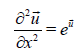This method could be applied towards the Navier-Stokes equations from a classical geometric theoretical perception of differential equations developed by a number of mathematicians including Darboux . The reason for using this method is to find general algorithmic approaches towards the derivation of possible solutions .

Another concept to study exists within the integrability of Dispersive Wave Equations. There is a method within the integrable wave equations called the Korteweg-de Vries method or KdV. There is a general equation for possible integration to yield,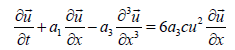where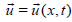[1,2]. This method of integrability is mentioned because it may have an implication of a correlation to the Navier- Stokes equations. The Korteweg-de Vries method of integrability may have a specific correlation to the next method I will discuss.

There is method for non-linear partial differential equations titled the Adomian of summation. Summation methodology is another way to integrate an equation depending on the necessity for it . There could exist a two-dimensional solution for vector field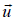in the Navier- Stokes equation. This solution may be calculated by taking the form of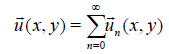with a nonlinear term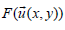by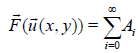Where Ai are Adomian polynomials generated for all general forms of nonlinearity . Does the summation of the vectorimply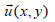to exist?

We now go back to the concept of Korteweg-de Vries equation and integrability analysis of it’s inverse. There is a kdV nonlinear dynamical system to yield,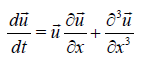Where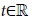is an evolution parameter and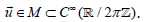The vector field being a proper subset is an implication towards the given divergent-free vector field on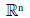in the existence of Navier- Stokes equations. The vector fieldis also said to be an element of an infinite-dimensional periodic functional manifold, hence the M. Using this method, the elementcan be integrated such that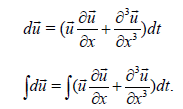Lastly, I want to discuss a specific concept called Kink Waves. These waves are formally defined as traveling waves which rise or descend from one asymptotic form to another . It is said that one of these solutions approaches or converges to a constant. In the concept of dissipative waves, there is an equation by Burger to show,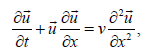Where v is the given viscosity. Since we are dealing with a viscosity and traveling waves, the Burger’s equation may have a direct correlation as to how the Navier-Stokes equations were developed. The Burger’s equation may also imply future solutions using integrability methodologies.

#### References

Select your language of interest to view the total content in your interested language

### Article Usage

• Total views: 8855
• [From(publication date):
September-2016 - Feb 22, 2020]
• Breakdown by view type
• HTML page views : 8707Can't read the image? click here to refresh